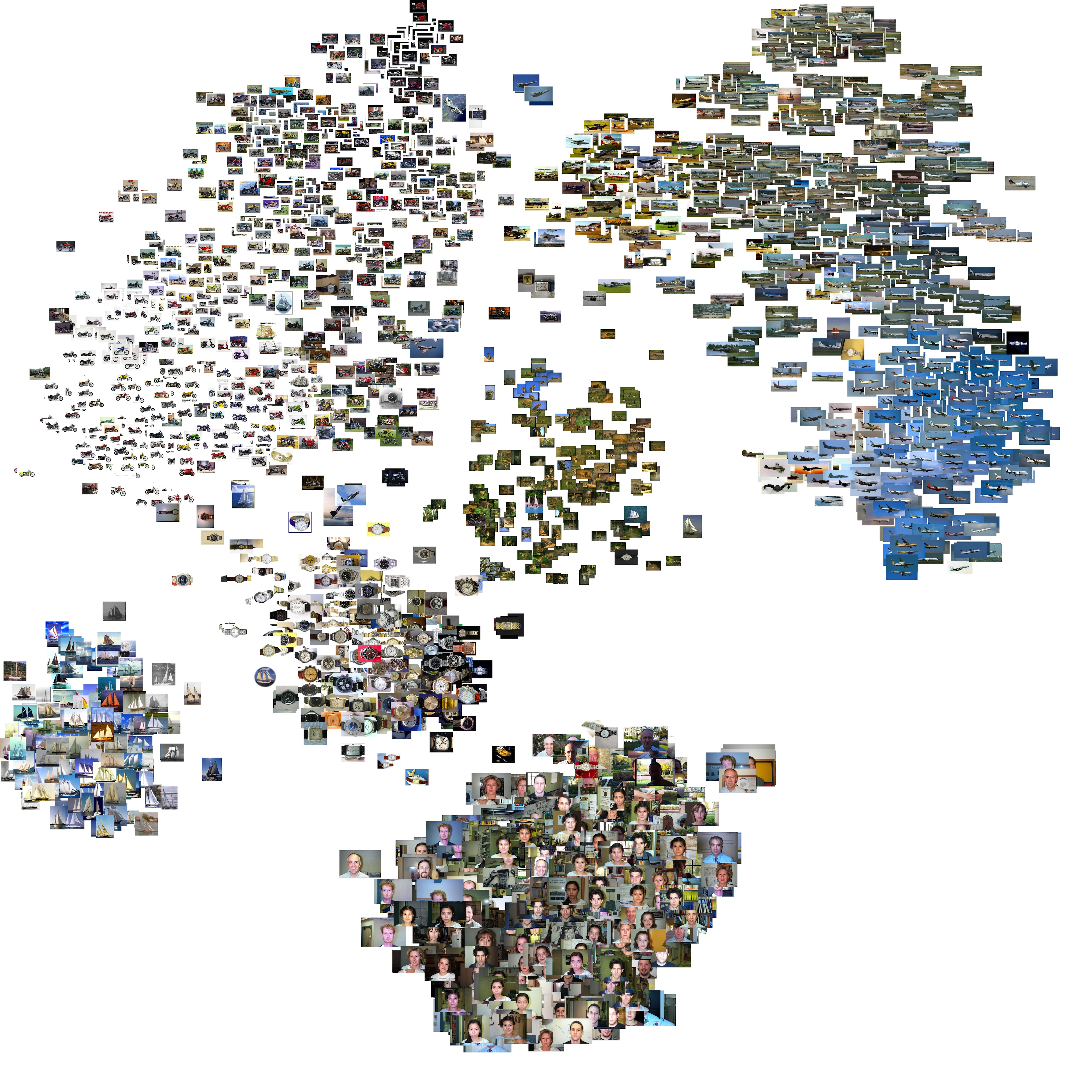Need help with tsne-js?
Click the “chat” button below for chat support from the developer who created it, or find similar developers for support.scienceai218 Stars37 ForksApache License 2.038 Commits7 Opened issues#### Description

t-distributed stochastic neighbor embedding (t-SNE) algorithm implemented in JavaScript!
?

#### Need anything else?# t-SNE.js

t-distributed stochastic neighbor embedding (t-SNE) algorithm implemented in JavaScript

• Runs in the browser (also runs in Web Workers)

• Runs in node.js

• Uses efficient in-place matrix operations via ndarray

• Follows closely the API of scikit-learn, allowing specification of perplexity and early exaggeration factor, among other parameters.

INTERACTIVE DEMO

### Background

t-SNE is a powerful manifold technique for embedding data into low-dimensional space (typically 2-d or 3-d for visualization purposes) while preserving small pairwise distances or local data structures in the original high-dimensional space. In practice, this results in a much more intuitive layout within the low-dimensional space as compared to other techniques. The low-dimensional embedding is learned by minimizing the Kullback-Leibler divergence between the pairwise-similarity probability distribution over the original data space and distribution over the embedding space.

An important note is that the objective function is non-convex with numerous local minima, and thus the results are non-deterministic. There are a few model parameters which influence the learning and optimization process. Selecting appropriate parameters for the input data can significantly improve the chances the model converge on good solutions.

Currently implemented is the exact fomulation, which has computational complexity O(dN^2), where d is the original dimensionality of the data and N is the number of samples. Implementation of the O(dN*logN) Barnes-Hut approximation variant is planned (contributions welcome!).source

### Usage

Can be run in node.js or the browser. In the browser, should ideally be run in a web worker.

###### node.js
```\$ npm install tsne-js --save
```
```import TSNE from 'tsne-js';

let model = new TSNE({
dim: 2,
perplexity: 30.0,
earlyExaggeration: 4.0,
learningRate: 100.0,
nIter: 1000,
metric: 'euclidean'
});
// inputData is a nested array which can be converted into an ndarray
// alternatively, it can be an array of coordinates (second argument should be specified as 'sparse')
model.init({
data: inputData,
type: 'dense'
});
// `error`,  `iter`: final error and iteration number
// note: computation-heavy action happens here
let [error, iter] = model.run();
// rerun without re-calculating pairwise distances, etc.
let [error, iter] = model.rerun();
// `output` is unpacked ndarray (regular nested javascript array)
let output = model.getOutput();
// `outputScaled` is `output` scaled to a range of [-1, 1]
let outputScaled = model.getOutputScaled();
```

###### browser

Then it's the same API as above. A browser example using Web Workers is in the

`example/`
folder.
###### Model Parameters
• `dim`
: number of embedding dimensions, typically 2 or 3
• `perplexity`
: approximately related to number of nearest neighbors used during learning, typically between 5 and 50
• `earlyExaggeration`
: parameter which influences spacing between clusters, must be at least 1.0
• `learningRate`
: learning rate for gradient descent, typically between 100 and 1000
• `nIter`
: maximum number of iterations, should be at least 200
• `metric`
: distance measure to use for input data, currently implemented measures include
• `euclidean`
• `manhattan`
• `jaccard`
(boolean data)
• `dice`
(boolean data)

### Build

To run build yourself, for both the browser (outputs to

`build/tsne.min.js`
) and node.js (outputs to
`dist/`
):
```\$ npm run build
```

To build for just the browser, run

`npm run build-browser`
, and to build for just node.js, run
`npm run build-node`
.

### Tests

```\$ npm test
```

### References

The original paper on t-SNE:

```L.J.P. van der Maaten and G.E. Hinton.
Visualizing High-Dimensional Data Using t-SNE.
Journal of Machine Learning Research 9(Nov):2579-2605, 2008.
```

Paper on Barnes-Hut variant t-SNE:

```L.J.P. van der Maaten.
Accelerating t-SNE using Tree-Based Algorithms.
Journal of Machine Learning Research 15(Oct):3221-3245, 2014.
```